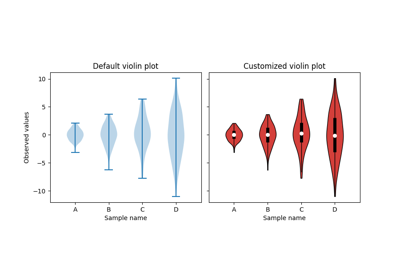# matplotlib.axes.Axes.violinplot¶

Axes.violinplot(self, dataset, positions=None, vert=True, widths=0.5, showmeans=False, showextrema=True, showmedians=False, points=100, bw_method=None, *, data=None)[source]

Make a violin plot.

Make a violin plot for each column of dataset or each vector in sequence dataset. Each filled area extends to represent the entire data range, with optional lines at the mean, the median, the minimum, and the maximum.

Parameters: dataset : Array or a sequence of vectors. The input data. positions : array-like, default = [1, 2, ..., n] Sets the positions of the violins. The ticks and limits are automatically set to match the positions. vert : bool, default = True. If true, creates a vertical violin plot. Otherwise, creates a horizontal violin plot. widths : array-like, default = 0.5 Either a scalar or a vector that sets the maximal width of each violin. The default is 0.5, which uses about half of the available horizontal space. showmeans : bool, default = False If True, will toggle rendering of the means. showextrema : bool, default = True If True, will toggle rendering of the extrema. showmedians : bool, default = False If True, will toggle rendering of the medians. points : scalar, default = 100 Defines the number of points to evaluate each of the gaussian kernel density estimations at. bw_method : str, scalar or callable, optional The method used to calculate the estimator bandwidth. This can be 'scott', 'silverman', a scalar constant or a callable. If a scalar, this will be used directly as kde.factor. If a callable, it should take a GaussianKDE instance as its only parameter and return a scalar. If None (default), 'scott' is used. result : dict A dictionary mapping each component of the violinplot to a list of the corresponding collection instances created. The dictionary has the following keys: bodies: A list of the matplotlib.collections.PolyCollection instances containing the filled area of each violin. cmeans: A matplotlib.collections.LineCollection instance created to identify the mean values of each of the violin's distribution. cmins: A matplotlib.collections.LineCollection instance created to identify the bottom of each violin's distribution. cmaxes: A matplotlib.collections.LineCollection instance created to identify the top of each violin's distribution. cbars: A matplotlib.collections.LineCollection instance created to identify the centers of each violin's distribution. cmedians: A matplotlib.collections.LineCollection instance created to identify the median values of each of the violin's distribution.

Notes

Note

In addition to the above described arguments, this function can take a data keyword argument. If such a data argument is given, the following arguments are replaced by data[<arg>]:

• All arguments with the following names: 'dataset'.

Objects passed as data must support item access (data[<arg>]) and membership test (<arg> in data).

## Examples using matplotlib.axes.Axes.violinplot¶Violin plot customization